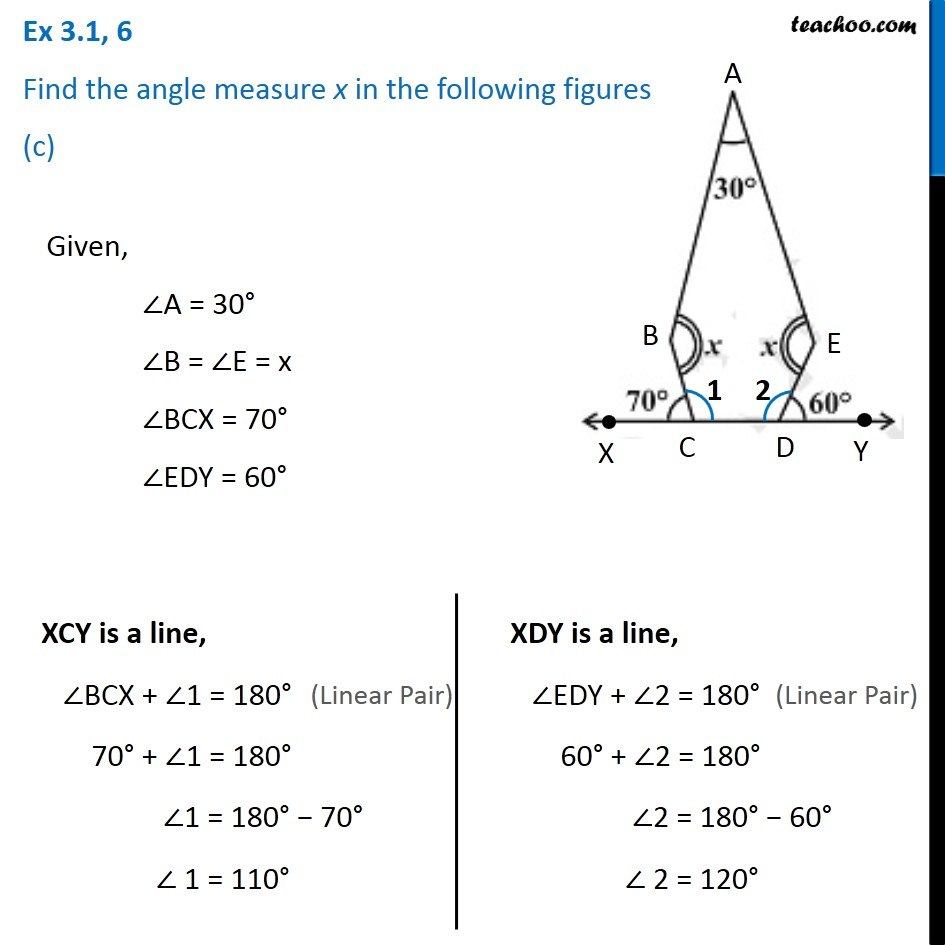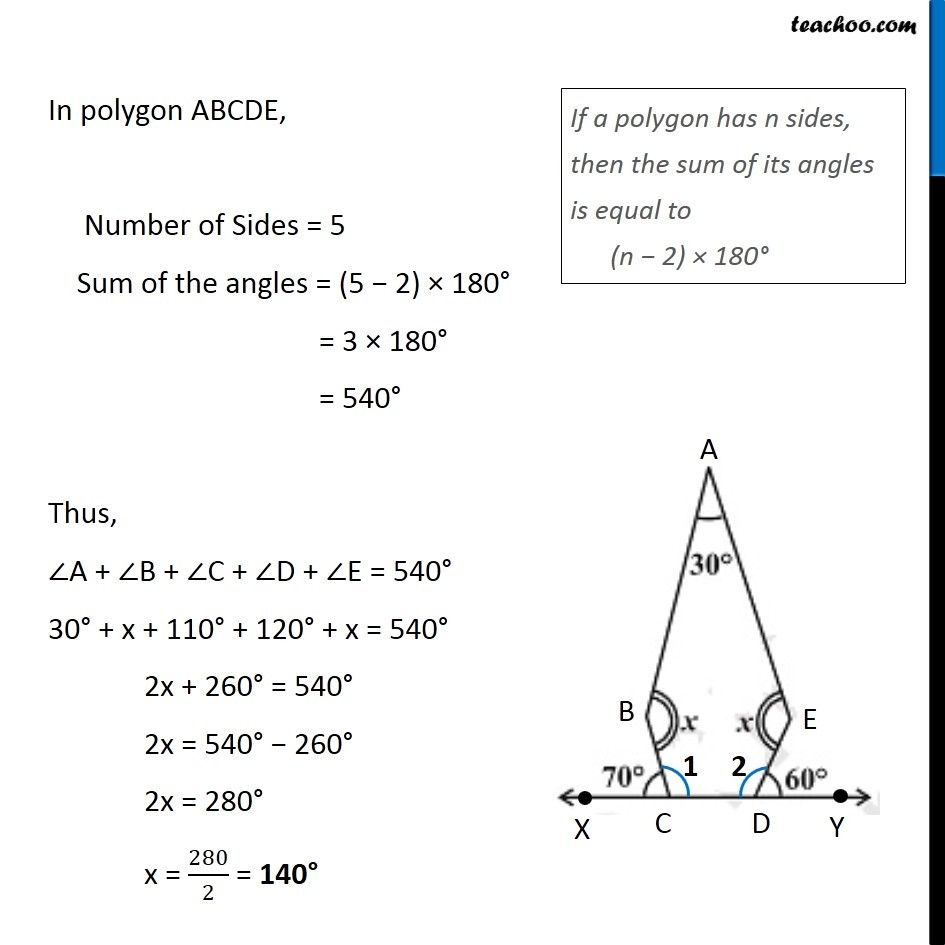Ex 3.1

Chapter 3 Class 8 Understanding Quadrilaterals
Serial order wiseLearn in your speed, with individual attention - Teachoo Maths 1-on-1 Class

### Transcript

Ex 3.1, 6 Find the angle measure x in the following figures (c)Given, ∠A = 30° ∠B = ∠E = x ∠BCX = 70° ∠EDY = 60° XCY is a line, ∠BCX + ∠1 = 180° 70° + ∠1 = 180° ∠1 = 180° − 70° ∠ 1 = 110° XDY is a line, ∠EDY + ∠2 = 180° 60° + ∠2 = 180° ∠2 = 180° − 60° ∠ 2 = 120° In polygon ABCDE, Number of Sides = 5 Sum of the angles = (5 − 2) × 180° = 3 × 180° = 540° Thus, ∠A + ∠B + ∠C + ∠D + ∠E = 540° 30° + x + 110° + 120° + x = 540° 2x + 260° = 540° 2x = 540° − 260° 2x = 280° x = 280/2 = 140° If a polygon has n sides, then the sum of its angles is equal to (n − 2) × 180°